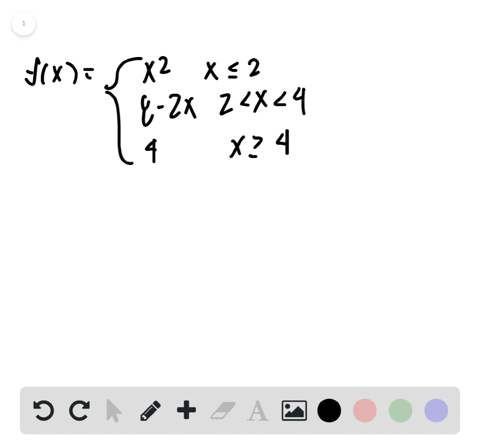🎉 The Study-to-Win Winning Ticket number has been announced! Go to your Tickets dashboard to see if you won! 🎉View Winning Ticket01:27
Problem 30

# Graphical Reasoning In Exercises 29 and 30 ,use the graph of the function $f$ to decide whether the value of the given quantity exists. If it does, find it. If not, explain why(a) $f(-2)$(b) $\lim _{x \rightarrow-2} f(x)$(c) $f(0)$(d) $\lim _{x \rightarrow 0} f(x)$(e) $f(2)$(f) $\lim _{x \rightarrow 2} f(x)$(g) $f(4)$(h) $\lim _{x \rightarrow 4} f(x)$

## Discussion

You must be signed in to discuss.

## Video Transcript

in this problem, we want to determine the following values. We want to find FF negative to the limit as X approaches. Negative too for the function half of zero and so on. So first worse will start with finding effort. Negative too. So if you look at our graph here and we look at the behavior of the function as excess approaching negative two from the left, our function values are approaching negative infinity. Sure. And we know those values are approaching negative affinity because we have a horizontal. I'm sorry. A vertical ask himto there and from the right were approaching positive infinity. So since those two right and left limits, do not agree. Say it. That's the limit as X approaches negative too for the function is equal. Teo does not exist now. If we look at effort negative too, we see that the function is not to find there they're simply a bird. A glass of talk. They're so we say out of two is undefined or in other words, negative too is not in the domain of the vex. Now we want to find out of syrup. If you look here at zero, we know that the function value at Cerrone is where the filled in dot is, which is appear at fourth. It's up of Sarah's. He goes for it. If you want that limit, it has X approaches. Zero We study the left and the right limits from the left. The limit is approaching this y value here, which is about 0.5. However, from the right, the function is approaching the Y value positive for Since the limits do not exist, we say since sorry. Since the limits do not green the left and right limits, we say that the limit does not exist. Now we want to find out of two. While the limit seems to exist here as both the left and right limits are approaching, 0.5 f of two is not find. So we say that, too is undefined. I two is not from the domain and like we just saw the limit as exit Purchase two is equal to 0.5. Now we want a full force. If we look over at four, we see the film in dot is at two so before is equal to two. But if we look at the limit The limit from the left appears to be positive. Infinity and the limit from the right. However, both limits are not finite values. So in this case, we say the limit does not exist.